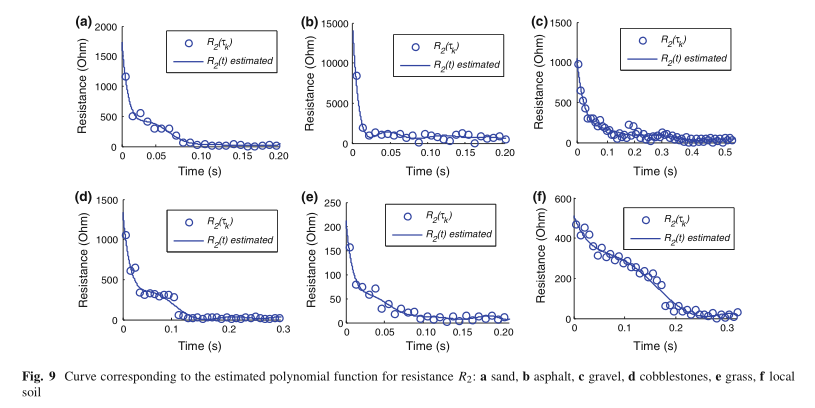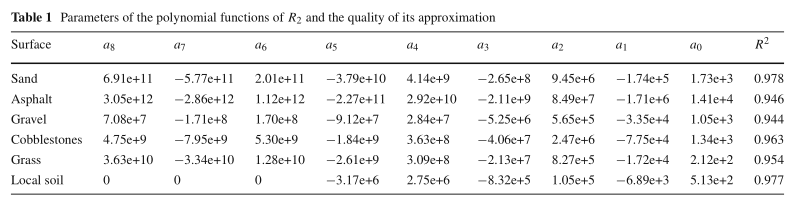# 1. Arc fault location: A nonlinear time varying fault model and frequency domain parameter estimation approach

## 1.2 引言

NAF表现出一些不常见的故障特征，当前的基于阻抗的最新配方并未考虑到这些特征，从而阻碍了它们的性能。 在制定问题时必须解决和考虑此类故障特征。从这个意义上讲，在基于阻抗的公式中必须考虑时变，非对称和非线性特性。

## 1.3 Modeling a NAF

Nonlinear arcing faults(NAF)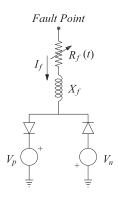Rf（t）的时变行为可以通过多项式函数来近似：

$R _ { f } ( t ) = \left\{ \begin{array} { l } { a _ { n } t ^ { n } + a _ { n - 1 } t ^ { n - 1 } + \cdots + a _ { 1 } t + a _ { 0 } , \text { for } t _ { fi } \leq t \leq t _ { ss } } \\ { a _ { 0 } , \text { for } t > t _ { ss } } \end{array} \right. \tag{1}$

# 2. A Modeling Method of a High Impedance Fault in a Distribution System Using Two Series Time-Varying Resistances in EMTP

## 2. HIF故障建模

### A. 高阻故障的特征

KEPCO在22,9（kV）配电系统上进行了HIF实验。 实验总数为32，采样频率为10 kHz。 但是，实验数据是在故障分支而不是中继点处测量的，这意味着在测量数据中不包含有关载荷的信息。

### B. HIF模型

$R ( t ) = R _ { 1 } ( t ) + R _ { 2 } ( t ) \tag{1}$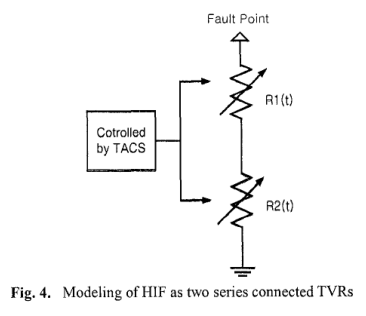$i ( t ) = i _ { n } + \frac { i _ { n + 1 } - i _ { n } } { v _ { n + 1 } - v _ { n } } \times ( v ( t ) - v _ { n } ) \tag{2}$

$R _ { 1 } ( t ) = \frac { v ( t ) } { i ( t ) } = \frac { v ( t ) } { i _ { n } + \frac { i _ { n + 1 } - i _ { n } } { v _ { n + 1 } - v _ { n } } \times ( v ( t ) - v _ { n } ) } \tag{3}$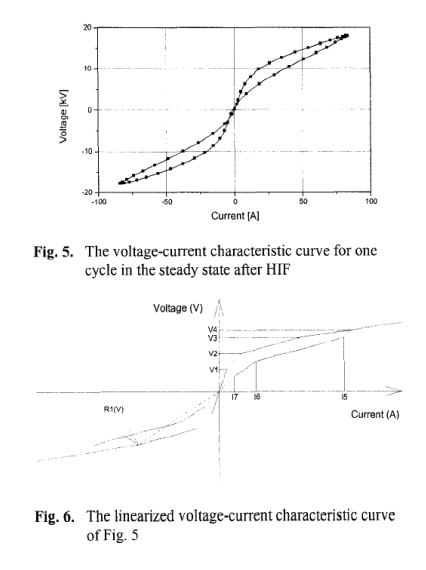# 3. High Impedance Faults: From Field Tests to Modeling

dos Santos W C, de Souza B A, Brito N S D, et al. High impedance faults: From field tests to modeling[J]. Journal of Control, Automation and Electrical Systems, 2013, 24(6): 885-896.

## 3.3 高阻模型综述

### 3.3.1 高阻模型的主要特征

HIF的主要特征是故障电流的幅值低，在大多数情况下，该幅值与由其他现象（例如负载通电/断电和电容器组切换）产生的电流大小相同（Wester 1998）。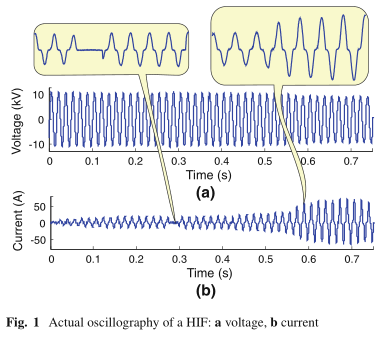### 3.3.2 高阻电弧模型综述

#### 模型1： Sharaf and Wang (2003)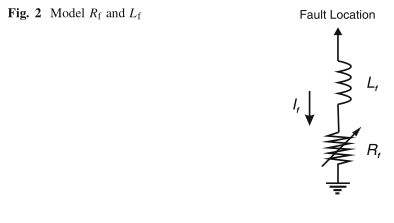R f的值计算如下：

$R _ { f } = R _ { f 0 } \cdot ( 1 + \alpha \cdot ( \frac { i _ { f } } { i _ { f 0 } } ) ^ { \beta } ) \tag{1}$

#### 模型2：Emanuel et al. (1990)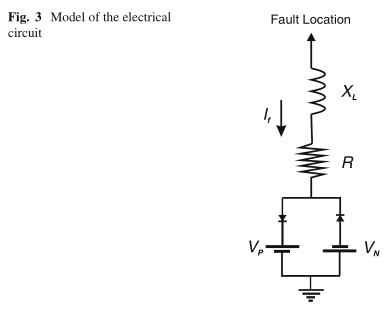#### 模型3：Nam et al. (2001)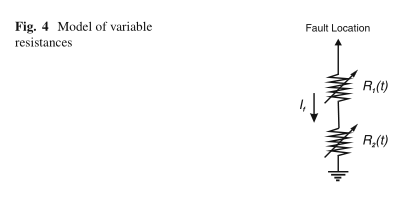## 3.4 高阻电弧模型建议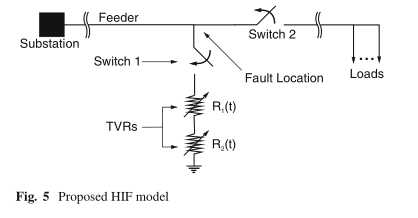• TACS控制的串联两次时变电阻（TVR），例如Nam等人提出的。 （2001）。 电阻R 1：模拟非线性和不对称的特性。 电阻R 2：模拟堆积和肩部现象。

• 在可变故障电阻模型中添加了两个时间控制的开关： 开关1：将电阻连接到故障点并启动故障。 开关2：连接到故障点的下游，模拟导体的断开。

HIF的某些诊断方法在其算法中考虑了电导体的断裂（Malagodi 1997）。此故障会导致故障点下游的电压明显失衡。 插入开关2可以考虑馈线末端的这种现象。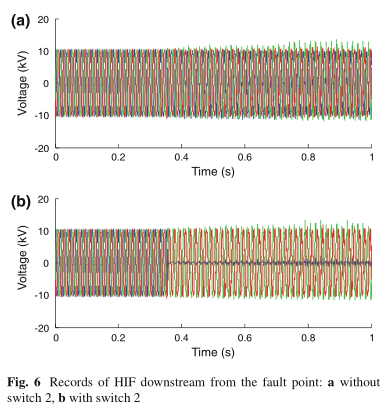Nam等人计算出与故障间隔电压相对应的电流。 （2001）：

$i ( t ) = \left\{ \begin{array} { c l } { i _ { n } + \frac { i _ { n + 1 } - i _ { n } } { v _ { n + 1 } - v _ { n } } \times \Delta v , } & { \text { se } v _ { n } < v ( t ) < v _ { n + 1 } } \\ { i _ { n } , } & { \text { se } v ( t ) = v _ { n } } \end{array} \right. \tag{2}$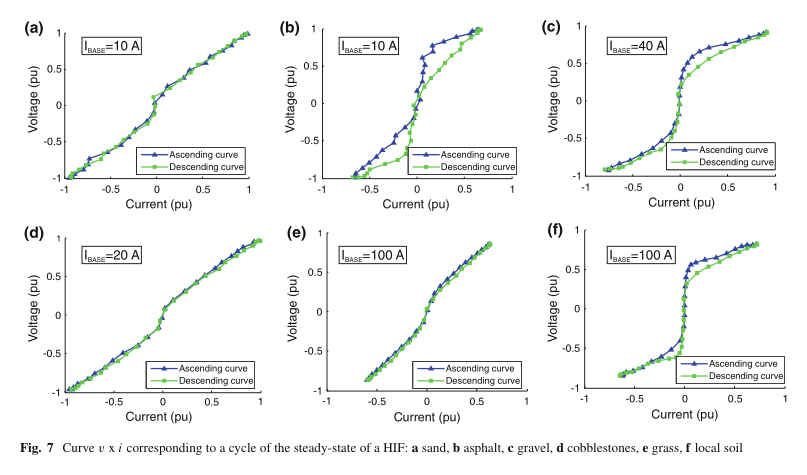$R _ { 1 } ( t ) = \frac { v ( t ) } { i ( t ) } = \frac { v ( t ) } { i _ { n } + \frac { i _ { n + 1 } - i _ { n } } { v _ { n + 1 } - v _ { n } } \times ( v ( t ) - v _ { n } ) } \tag{3}$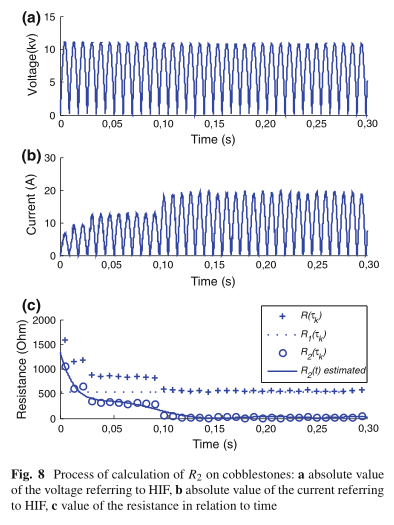HIF中的电流主要具有电阻特性。因此，将电流与电压同相考虑（Nam等人，2001年）。 因此，总电阻（R = R 1 + R 2）可以通过将v的值除以i来直接获得。

$R _ { 2 } ( t ) = \left\{ \begin{array} { c c } { a _ { n } \cdot t ^ { n } + a _ { n - 1 } \cdot t ^ { n - 1 } + \dots + a _ { 1 } \cdot t + a _ { 0 } , } & { \text { set } < \Delta t } \\ { 10 ^ { - 5 } , } & { \text { se } t \geq \Delta t } \end{array} \right. \tag{4}$

$SSE = \sum _ { j = 1 } ^ { m } [ r _ { med } ( j ) - r _ { est } ( j ) ] ^ { 2 } \tag{5}$ $SST = \sum _ { j = 1 } ^ { m } [ r _ { [ \operatorname { med } } ( j ) - \overline { r } _ { med } ] ^ { 2 } \tag{6}$ $R ^ { 2 } = 1 - \frac { SSE } { SST } \tag{7}$